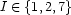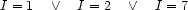## 6.2 Constraint Variables

Of particular relevance to this course are finite domain (FD) variables and finite set (FS) variables.

### 6.2.1 FD Variables

A FD variabledenotes an integer out of a finite domain of possible values. In the store, it is represented by a basic constraint of the form e.g.:This is essentially a form of disjunction. Its declarative semantics are simply:FD variables are a very economical and effective way to address certain forms of ambiguity arising in computational linguistics. Any finite collection of values/objects can be encoded as a finite domain: therefore an underspecified element of this collection can be represented by a FD variable. In Chapter 3, we illustrate this idea for agreement in German.

### 6.2.2 FS variables

A FS variabledenotes a finite set of integers. In the store it is represented by a basic constraint providing information about lower and upper bounds:Again, this a form of disjunction:may take as value any set that contains at least 1 and 7, and at most 1, 4, 5 and 7. Sets are incredibly useful in computational linguistic applications: (1) they permit elegant and succinct axiomatizations, (2) these axiomatizations are also efficient constraint programs.

Denys Duchier
Version 1.2.0 (20010221)